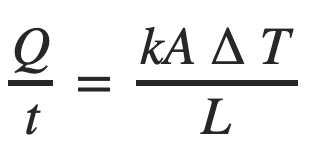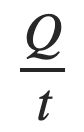# What is Thermal Conductivity?

Thermal conductivity is a measure of the ability of a certain material to transfer or conduct heat. Conduction occurs when a temperature gradient is present across the material. Its units are (W/mK) and is denoted either by λ or k.

The second law of thermodynamics specifies that heat will always flow from a higher temperature to a lower temperature.

The thermal conduction equation is calculated using the following formula:represents the heat energy transferred per unit time through the material. This is expressed in joules per second or watts.

• k is the thermal conductivity constant.
• A is the surface area through which heat energy flows and is measured in m2.
• ∆T is the difference in temperature measured in kelvin.
• L refers to the thickness of the material, through which heat is transferred and is measured in m.
• In order to calculate the thermal conductivity constant, one would use the following equation:The thermal conductivity of a specific material is dependent on its density, moisture content, structure, temperature and pressure.

## How is it measured?

Some common techniques to measure thermal conductivity are:

### The guarded hot plate method:

The guarded hot plate method is a widely utilised steady state technique to measure thermal conductivity. The material that needs to be tested is positioned between a hot plate and a cold plate. The parameters used to calculate thermal conductivity are the steady state temperatures, the heat used for the warmer plate, and the thickness of the material. It can be used for temperature ranges of 80 – 1500 K and for materials such as plastic, glass and insulation samples. It is very accurate but takes a significant amount of time to conduct the test.

### The hot wire method:

The hot wire method is a transient technique and can be used to determine the thermal conductivity of liquids, solids and gases. The standard hot wire method utilised for liquids involves a heated wire being placed in the sample. The thermal conductivity is determined by comparing the plot of the temperature of the wire against the logarithm of time, when the density and capacity are given.

In the case of solids, a slight modification to this method is needed whereby the hot wire is supported on a backing so that there is no penetration of the solid. It works in the temperature range of 298 – 1800 K and is a fast, accurate technique but has a key limitation in that it only works on low-conductivity materials.

### The comparative cut bar method:

The comparative cut bar method is a steady state technique and can be used to test metals, ceramics and plastics. Heat flux is passed through samples whose the thermal conductivity is known and unknown, consequently a comparison can be made between the thermal gradients. It works in the temperature range of 293 – 1573 K but the measurement is relatively uncertain.

### The laser flash method:

The laser flash method is a transient technique, in which a laser pulse delivers a short heat pulse to the front end of a sample and the temperature change is measured at the back end of the sample. It works in the temperature range of 373 – 3273 K and can be used for both solids and liquids. It has the advantage of being fast and has a high accuracy but is quite expensive.

### The heat flow meter method:

The heat flow meter method is a steady state technique and is similar to the guarded hot plate method except that heat flux transducers are used to measure the heat flow through the specimen, rather than a main heater. The heat flux is determined based on looking at the drop in temperature within a thermal resistor. Heat flow meters are used for the 373 – 573 K temperature range and can be used for plastics, ceramics, insulation material and glass. The main advantage of a heat flow meter is that they are relatively simple to set up, however the measurement is not particularly accurate.

## Which materials have the highest/lowest thermal conductivity?

As expected, materials that conduct heat well, such as metals, have a higher thermal conductivity constant than materials which do not conduct heat as effectively, such as polymers and wood.

Within the metals group, silver has the highest thermal conductivity constant and bismuth has the lowest.

Thermal conductivity of non-metallic liquids is much lower than the thermal conductivity of metals, and the lowest thermal conductivity is seen in gases. Among the gases, hydrogen and helium have relatively high thermal conductivities.

## Which applications require high/low thermal conductivity?

Phase change materials used for thermal energy storage applications such as heating and cooling systems, need to have a high thermal conductivity in order to maximise efficiency, whereas materials of low thermal conductivity are generally used for thermal insulation.

## Sources

• Materials that conduct heat well, such as metals, have a higher thermal conductivity constant than materials such as polymers and wood
• Within the metals group, silver has the highest thermal conductivity constant and bismuth has the lowest
• The lowest thermal conductivity is seen in gases